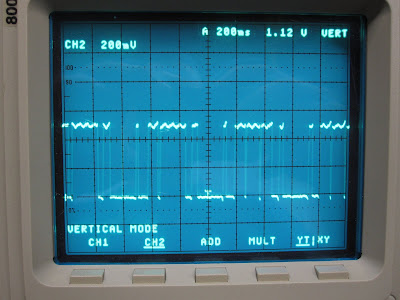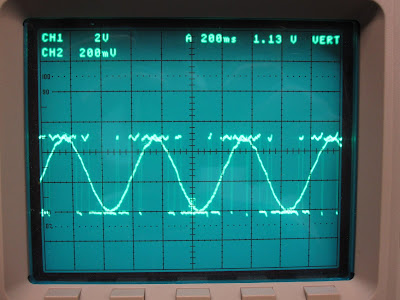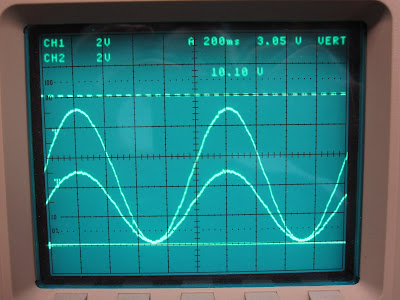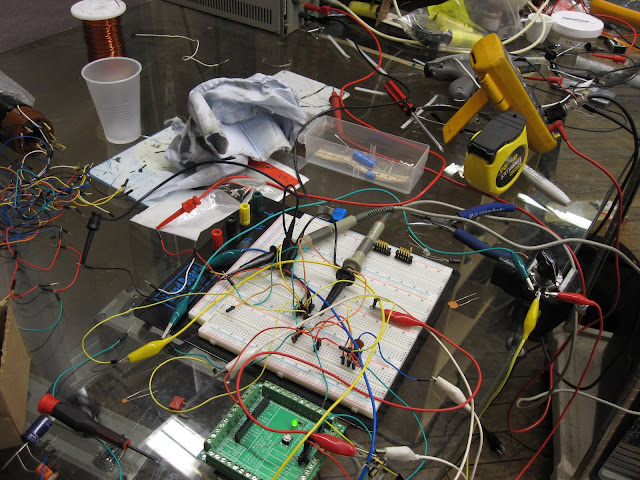## Arduino Controls 30,000 Volts

10 09 2011

Today I made arduino control 30,000 volts.

My arduino has 3 channels of analog output 0 to 5 volt.

For testing I used this sin wave generator sketch:

int pwmPin = 9; // output pin supporting PWM

void setup(){

pinMode(pwmPin, OUTPUT); // sets the pin as output

}

void loop(){

float something = millis() / 1000.0;

int value = 127.5 + 127.5 * sin( something * 2.0 * PI );

analogWrite(pwmPin,value);

}

This generates a lazy 2 Hz sin wave.

But the output is not really analog, it’s pulse width modulation(PWM):This tutorial shows how to smooth out  PWM using a low pass filter. My low pass filter used 6kΩ resistor and 4.7 µF @ 45V capacitor.

Here we have the raw PWM output superimposed with the filtered output:Looks good!

Now we just add the voltage doubling op-amp circuit I made previously, and BOOM:This shows the source signal and the voltage doubled signal.

Sweet! Now we can control the 30,000 volt glassman power supply.

Here the arduino is sending a slow sin wave to the glassman’s voltage control:

The Glassman’s slew rate is really slow without a load.

Here is the setup: